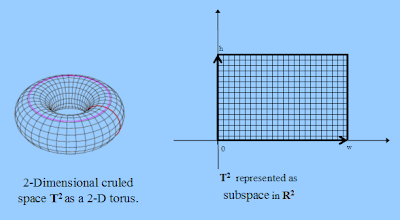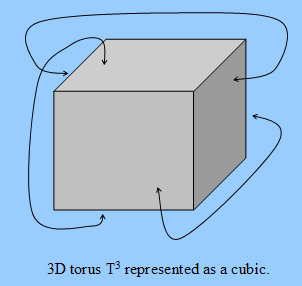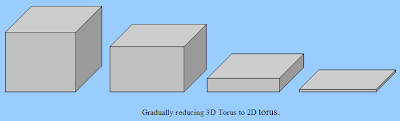## Friday, July 20, 2007

### RPM, Curled Space and Dimensionality Reduction

The Relational Perspective Map (RPM) uses finite curled spaces as information space to map high dimension data. The curled space has caused some uneasiness for users without related background. In this blog I will explain a little about the curled spaces and describe an advantage of the curled spaces over the normal (infinite) Euclidean space.

First, in order to understand the curled space let us compare the 1-dimensional curled space with the 1-dimensional Euclidean space. The 1-dimensional Euclidean space (denoted as R) comprises all real numerical values from negative infinite to positive infinite. Graphically, the 1-dimensional Euclidean space can be depicted by a straight line that extends in both directions to the infinite as be shown by the first map in the following picture.The curled 1-dimensional space, denoted as T, is graphically equivalent to a circle with a finite size as show in the middle map in above picture. To illustrate the difference between R and T it would be helpful to image an ant living in the spaces. An ant living in R, can walk in both directions indefinitely without coming back to the same spot. An ant living in T can also walk in both directions indefinitely, but it will come back to the same spot after a finite time of walking.

The curled space T can also represented as a segment of R of a finite length, say w, as be shown in the right map in above picture. In this representation, the begin and the end of the segment has to be considered as stuck together. That means, an imaginative ant walking on the segment can somehow be beamed from one end to anther end in no time. Analytically, T is represented by an interval [0, w]. The distance between two points a and b in T has to be defined differently as in R, though. One simple definition for the distance between two points a and b in T is as follows:
d(a, b) := min{|a-b|, w-|a-b|}

The analytically representation ([0,w], d) of T has the advantage over the graphical representation that it can be easily extended to high dimensional cases as we will see below.

The two dimensional curled space T2 is graphically equivalent to the surface of a torus as depicted in the left map of the following picture. The torus surface can also be understood as the trace of a circle (i.e. T) moving along another circle. Analogously, we can represent the T2 as rectangle of the width w and height h embedded in the 2-dimensional Euclidean space R2 as depicted in the right map of the following picture.The distance calculation on T2 becomes somewhat more complicated. If we define the distance based on the first graphical representation, we would have to use path integral in the R3 which is certainly not trivial. Based on the second representation (as shown in the right map of the picture above) we can have a much simple distance function as follows:
d((x1,y1), (x2,y2)) := min{|x2-x1|, w-|x2-x1|} + min{|y2-y1|, h-|y2-y1|}
This distance function is equivalent to any other valid distance function on T2 in the sense that two closely located points with respect to this distance function will also be close to each other with respect to any other valid distance function. This distance can be explained with an imaginative ant on the rectangle as follows: If we assume that the ant can only walk horizontally or vertically, and it can be beamed from any edge to its opposite edge in no time, Then the distance between two points a and b is the shortest walking distance for our ant to walk from a to b.
The 3-dimensional curled dimension T3 cannot be represented as a subspace of R3, but it can represented as a cubic whose opposite planes are identified with each other as depicted in the following picture:The distance function on T3 can be defined analogously as on T2 which we will omit here.
After explained the curled dimension we can now talk about the dimensionality reduction (DR). In general, DR means to squeeze data from high dimensional spaces into lower dimensional spaces. The lower dimensional representation of high dimensional data allows us to study the data by means of visualization, but the cost for DR is that we will lose some information which are present in the original high dimensional space.

One main challenge for DR algorithms is to preserve as much as possible relevant information. In order to do so, most DR methods define some kind of stress function that measures information lost of the lower dimensional representation. In this way, a DR algorithm is converted to an optimization algorithm that minimizes the stress function.
For any no-trivial minimization problem we all know that local minimum is a problem, and there more we squeeze the data, the worse the problem. One typical strategy to avoid local minimum is to introduce some kind of global permutation or variation, that doesn't always reduces the stress function, but might bridge us to a global minimum at the end. Genetic algorithm and simulated annealing, for instance, employ such strategy. The RPM algorithm also implicitly employs global strategy by using very large learning speed that vanishes gradually like the temperature in simulated annealing algorithm.
RPM algorithm also offers another strategy to avoid local minimum because of its use of curled finite dimensions. As mentioned above, there more we squeeze our data, the worse the local minimum problem will become. This also means that we can alleviate the local minimum problem by gradually squeeze data from high dimensional space to lower dimensional space.
More particularly, to create a 2-dimensional map for a dataset we can first map it first to 3-dimensional torus T3, then gradually reduces its height and ultimately change it to a 2-dimensional torus T2 as depicted in the following picture:The gradual dimensionality reduction is possible because the dimensions have finite size, so that we can change a dimension's size gradually to zero to effectively remove the dimension. In other words, we change our information space from dimension 3 to dimension 2.9, 2.8, 2.7, ... till 2.0. The partial dimension reflects the reduced size of the a selected curled dimension. More generally, we can use the same method to squezze a map from any high dimension space, dimension by dimension, into lower dimensional space.
With VisuMap software we can automated the gradual DR with a script. The following picture shows how RPM algorithm gradually squeezes a spherical dataset from T3 to T2. In this way, we can expect that the 2-dimensional map is much closer to the global minimum.